# Recursion Recursion What is recursion When one function

• Slides: 23RecursionRecursion What is recursion? When one function calls itself directly or indirectly. Gcd. Find largest integer d that evenly divides into p and q. base case reduction step, converges to base case public static int gcd(int p, int q) { if (q == 0) return p; else return gcd(q, p % q); } base case reduction step 23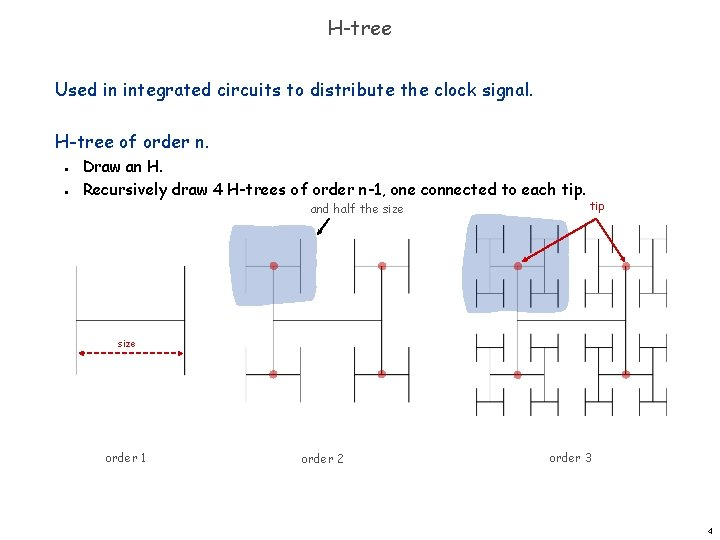H-tree Used in integrated circuits to distribute the clock signal. H-tree of order n. n n Draw an H. Recursively draw 4 H-trees of order n-1, one connected to each tip. and half the size tip size order 1 order 2 order 3 4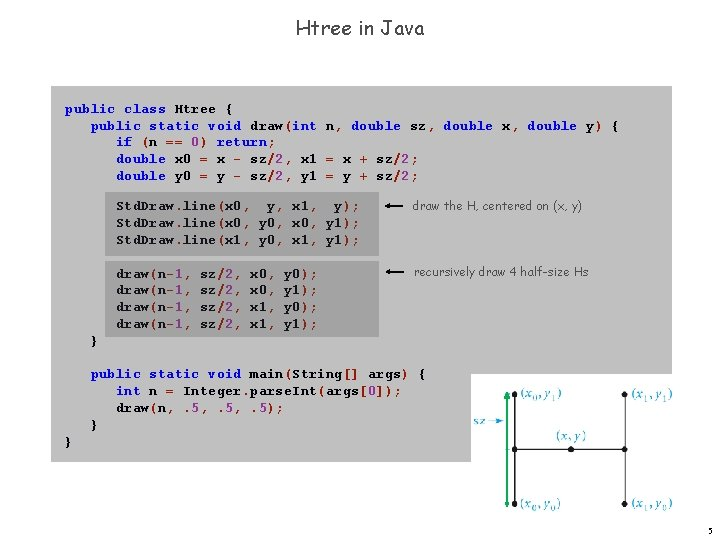Htree in Java public class Htree { public static void draw(int n, double sz, double x, double y) { if (n == 0) return; double x 0 = x - sz/2, x 1 = x + sz/2; double y 0 = y - sz/2, y 1 = y + sz/2; Std. Draw. line(x 0, y, x 1, y); Std. Draw. line(x 0, y 0, x 0, y 1); Std. Draw. line(x 1, y 0, x 1, y 1); draw the H, centered on (x, y) draw(n-1, recursively draw 4 half-size Hs sz/2, x 0, x 1, y 0); y 1); } public static void main(String[] args) { int n = Integer. parse. Int(args); draw(n, . 5, . 5); } } 5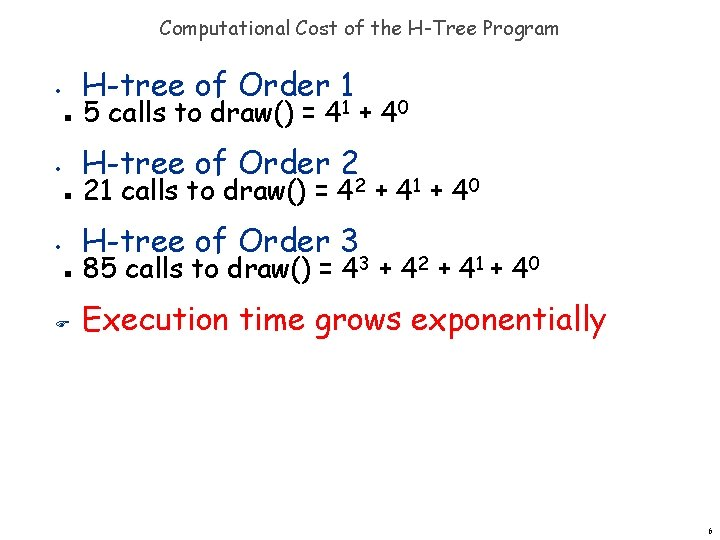Computational Cost of the H-Tree Program H-tree of Order 1 • n 5 calls to draw() = 41 + 40 H-tree of Order 2 • n 21 calls to draw() = 42 + 41 + 40 H-tree of Order 3 • n F 85 calls to draw() = 43 + 42 + 41 + 40 Execution time grows exponentially 6Towers of Hanoi http: //en. wikipedia. org/wiki/Image: Hanoiklein. jpg 7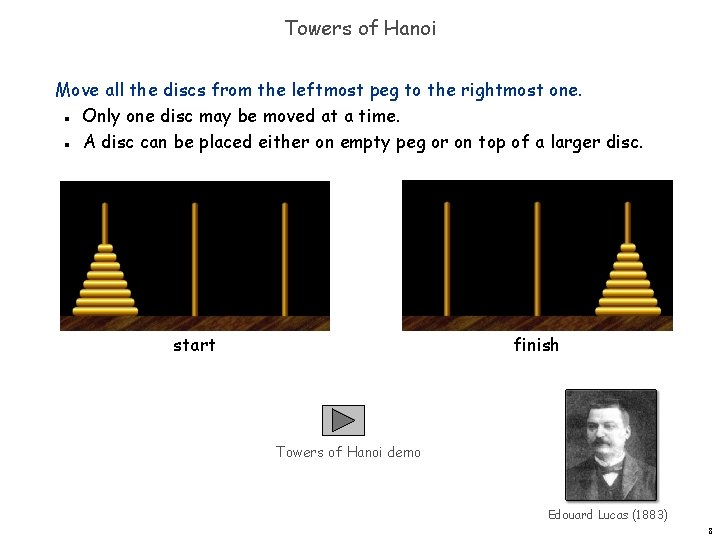Towers of Hanoi Move all the discs from the leftmost peg to the rightmost one. Only one disc may be moved at a time. A disc can be placed either on empty peg or on top of a larger disc. n n start finish Towers of Hanoi demo Edouard Lucas (1883) 8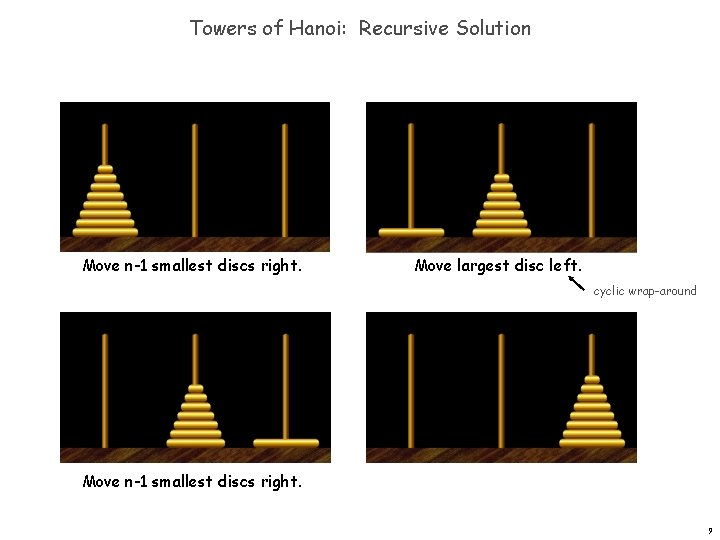Towers of Hanoi: Recursive Solution Move n-1 smallest discs right. Move largest disc left. cyclic wrap-around Move n-1 smallest discs right. 9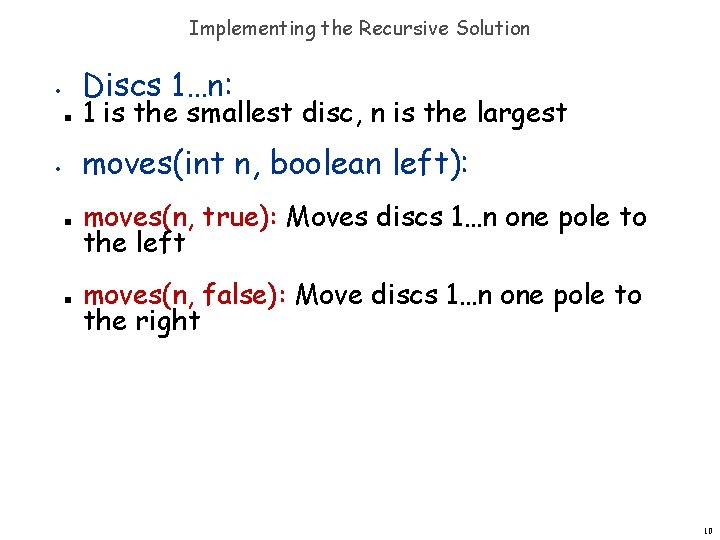Implementing the Recursive Solution Discs 1…n: • n 1 is the smallest disc, n is the largest moves(int n, boolean left): • n n moves(n, true): Moves discs 1…n one pole to the left moves(n, false): Move discs 1…n one pole to the right 10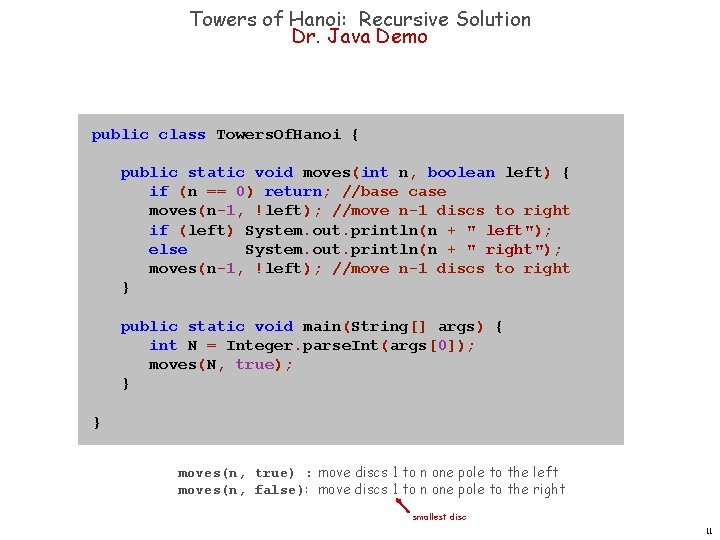Towers of Hanoi: Recursive Solution Dr. Java Demo public class Towers. Of. Hanoi { public static void moves(int n, boolean left) { if (n == 0) return; //base case moves(n-1, !left); //move n-1 discs to right if (left) System. out. println(n + " left"); else System. out. println(n + " right"); moves(n-1, !left); //move n-1 discs to right } public static void main(String[] args) { int N = Integer. parse. Int(args); moves(N, true); } } moves(n, true) : move discs 1 to n one pole to the left moves(n, false): move discs 1 to n one pole to the right smallest disc 11Recursive Function Call Tree On the Board 12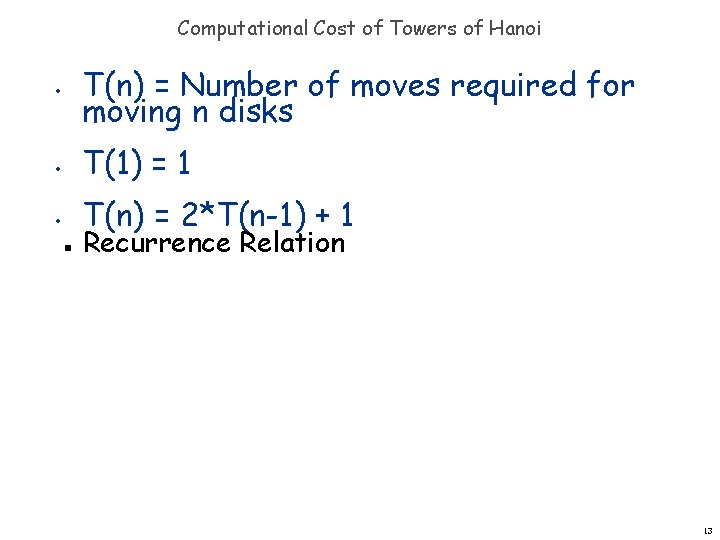Computational Cost of Towers of Hanoi • T(n) = Number of moves required for moving n disks • T(1) = 1 • T(n) = 2*T(n-1) + 1 n Recurrence Relation 13Computational Cost of Towers of Hanoi T(n) = 2*T(n-1)+1; T(1) = 1 • 2 Discs; T(2) = 3 moves • 3 Discs; T(3) = 7 moves • 4 Discs; T(4) = 15 moves • What is a closed-form expression for n discs? n F n Discs: T(n) = 2 n -1 Execution time grows exponentially 14So What if Time Grows Exponentially? Assume that it takes 1 second to make a single move: • n n • • 1 disc: 21 -1 = 1 second 10 discs: 210 -1 seconds ~ 17 minutes 20 discs: 220 -1 seconds ~ 12 days 30 discs: 230 -1 seconds ~ 34 years! Algorithms with exponential running times are no match even for the fastest computers for large values of n Can we implement a non-recursive solution? 15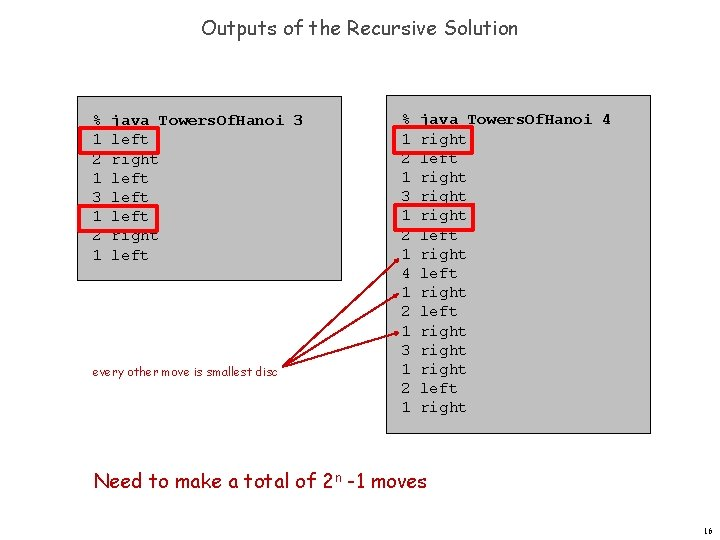Outputs of the Recursive Solution % 1 2 1 3 1 2 1 java Towers. Of. Hanoi 3 left right left every other move is smallest disc % 1 2 1 3 1 2 1 4 1 2 1 3 1 2 1 java Towers. Of. Hanoi 4 right left right right left right Need to make a total of 2 n -1 moves 16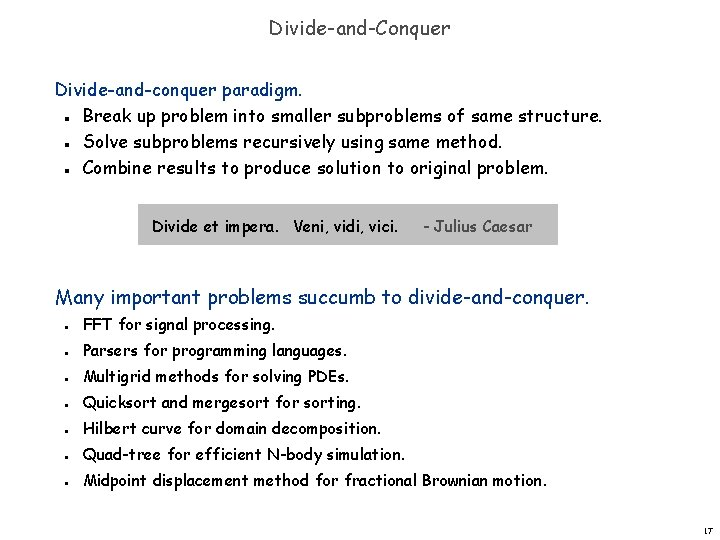Divide-and-Conquer Divide-and-conquer paradigm. Break up problem into smaller subproblems of same structure. Solve subproblems recursively using same method. Combine results to produce solution to original problem. n n n Divide et impera. Veni, vidi, vici. - Julius Caesar Many important problems succumb to divide-and-conquer. n n n n FFT for signal processing. Parsers for programming languages. Multigrid methods for solving PDEs. Quicksort and mergesort for sorting. Hilbert curve for domain decomposition. Quad-tree for efficient N-body simulation. Midpoint displacement method for fractional Brownian motion. 17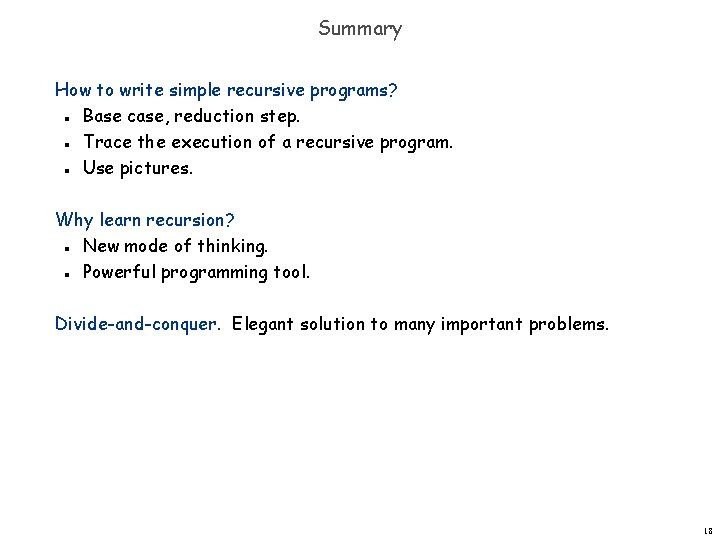Summary How to write simple recursive programs? Base case, reduction step. Trace the execution of a recursive program. Use pictures. n n n Why learn recursion? New mode of thinking. Powerful programming tool. n n Divide-and-conquer. Elegant solution to many important problems. 18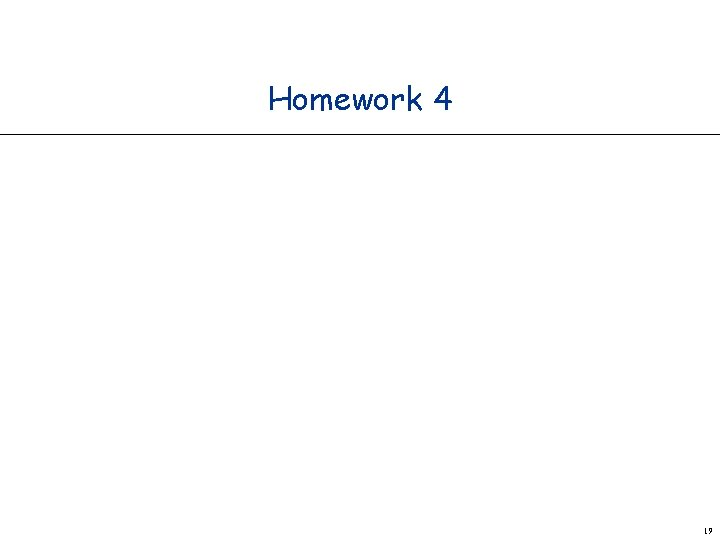Homework 4 19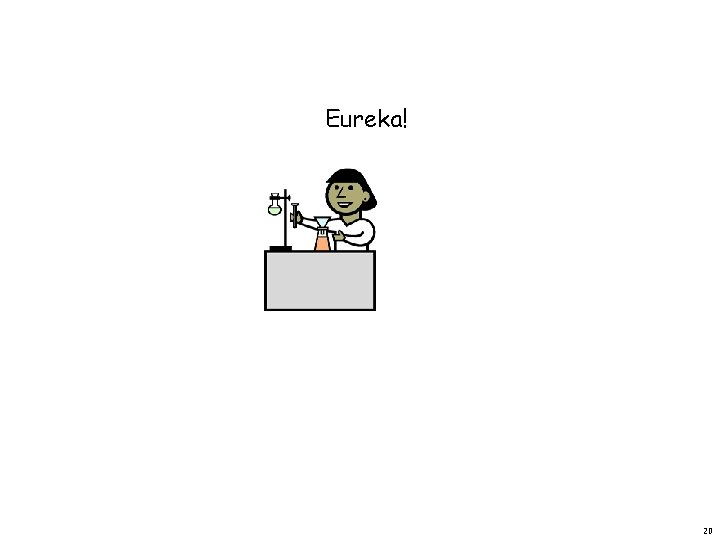Eureka! 20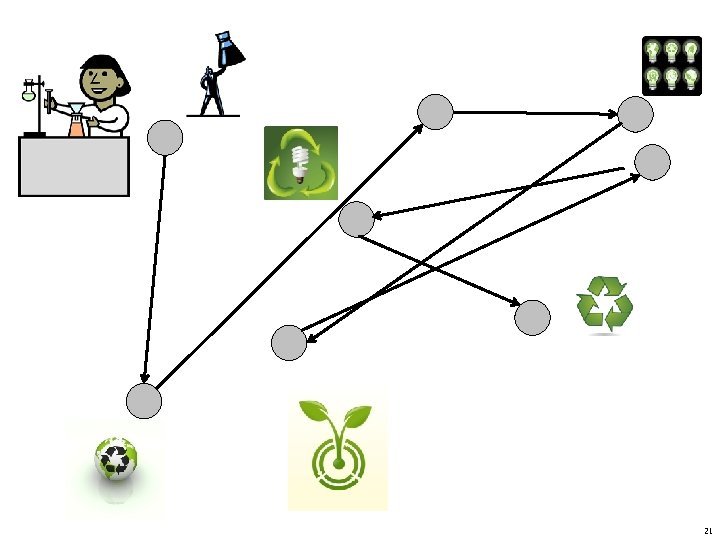2122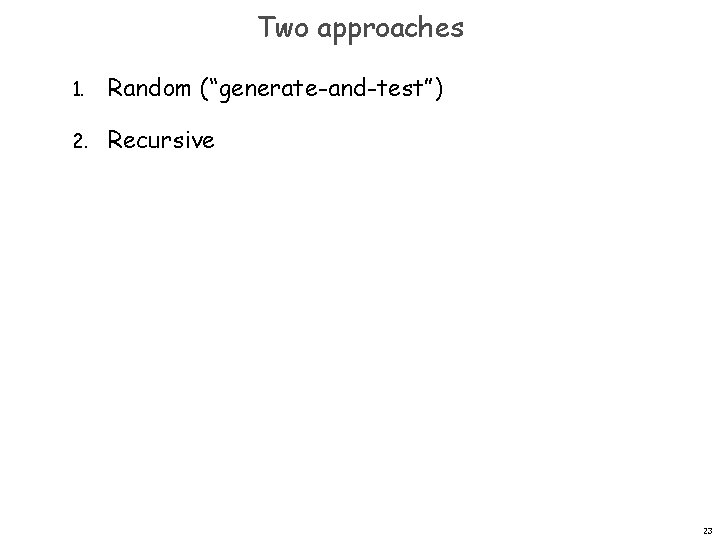Two approaches 1. Random (“generate-and-test”) 2. Recursive 23